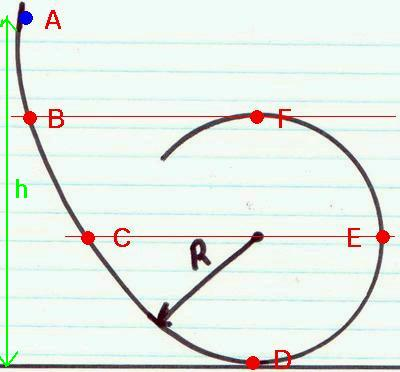# Conservation of energyA ball of mass $m=5$ kg is rolled, from rest, down a metal track, starting from point A as shown. Point A is $10$ meters above the ground, and the radius of the loop in the track is $R= 1$ meters. How fast is it moving at the point D?

×